• MATLAB绘制矢量场图的函数是quiver，利用help命令查看该函数的解释。 一般来说，输入参数可以是四个（x,y,u,v）,也可以是两个参数(u,v)，要求是这些参数都大小相同的矩阵。 注意：在输入参数只有两个(u,v)，...
Step 1

在MATLAB中绘制矢量场图的函数是quiver，利用help命令查看该函数的解释。
一般来说，输入参数可以是四个（x,y,u,v）,也可以是两个参数(u,v)，要求是这些参数都大小相同的矩阵。
注意：在输入参数只有两个(u,v)，实际上是默认[x,y]=meshgrid(0:length(u),0:length(u))。

Step 2

参数：（x,y）表示矢量的起点，,(u,v)表示矢量的大小和方向。
描述：1、数学上可以描述二元函数的梯度，梯度是一个矢量哦。

2、物理学上可以描述电场线、磁场线。
点电荷的电势：，电势的梯度实际上就是电场强度。

Step 3

例子1：描述圆锥的二元函数，矢量图表示梯度。
[x,y]=meshgrid(-2:0.1:2,-2:0.1:2);
z=sqrt(x.^2+y.^2);
mesh(x,y,z);
u=x./sqrt(x.^2+y.^2);
v=y./sqrt(x.^2+y.^2);
figure;
quiver(x,y,u,v,1);
三维图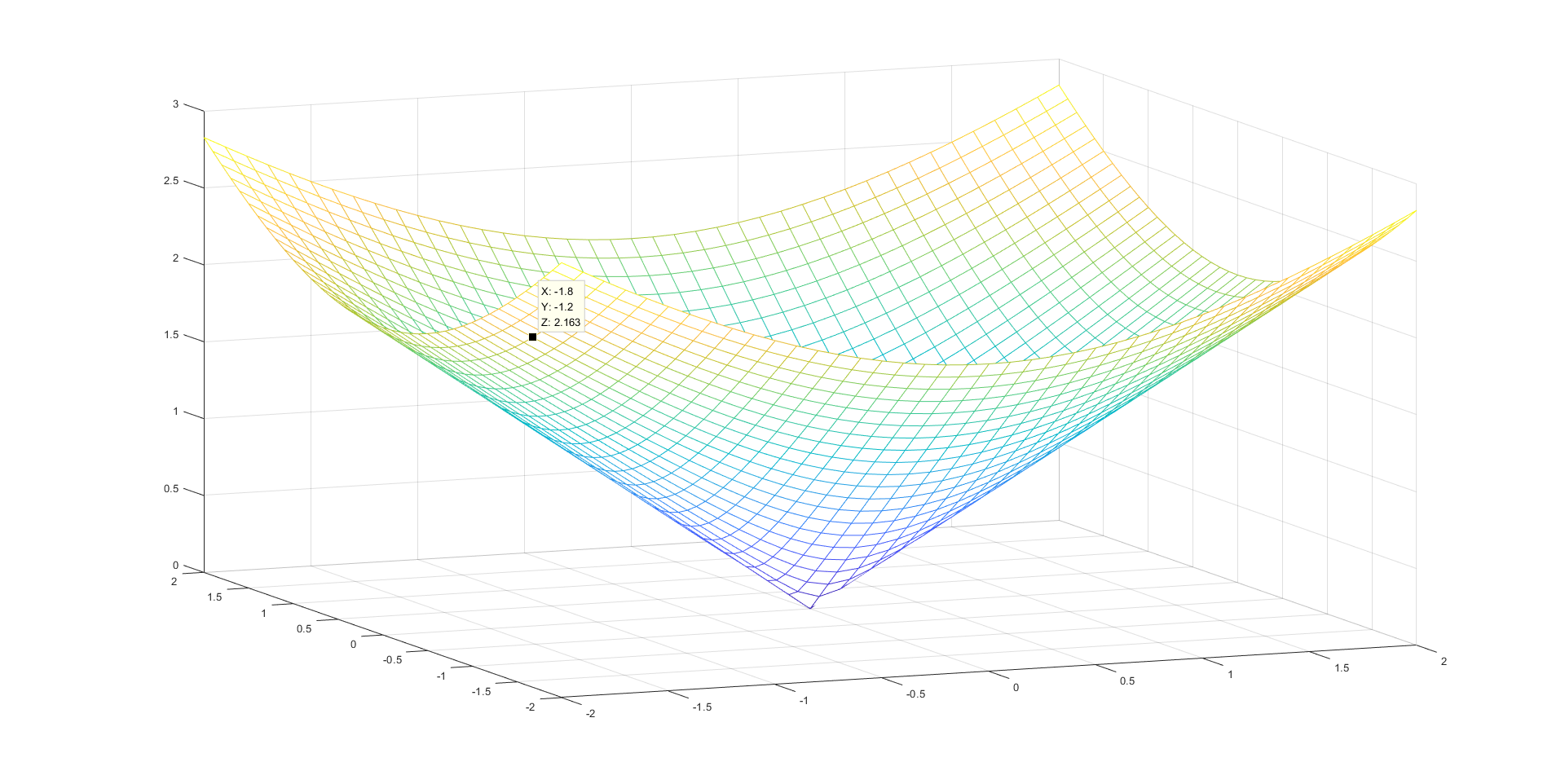矢量场图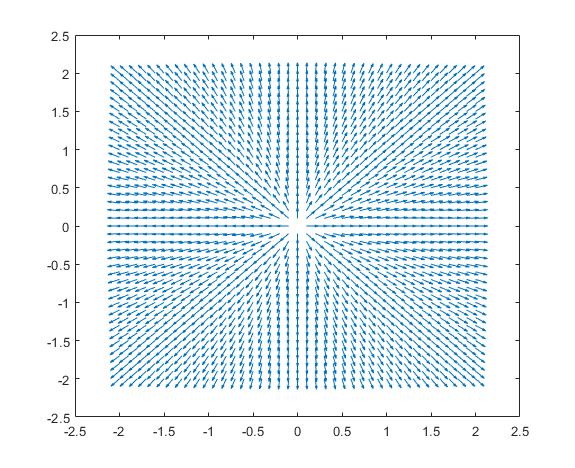例子2：Plot the gradient （梯度） of the function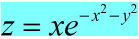.。
[X,Y] = meshgrid(-2:.2:2);
Z = X.*exp(-X.^2 - Y.^2);
//求梯度
figure
contour(X,Y,Z)//求等势线
hold on
quiver(X,Y,DX,DY)//求矢量图
hold off
三维图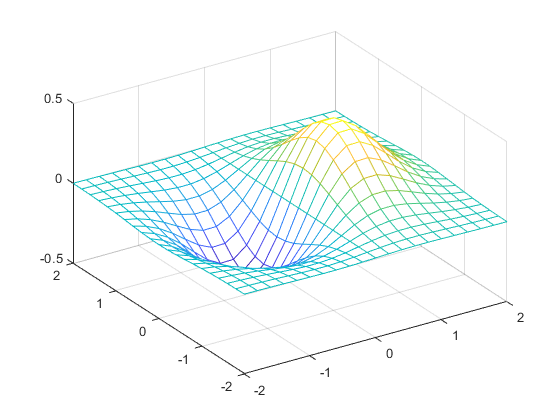矢量场图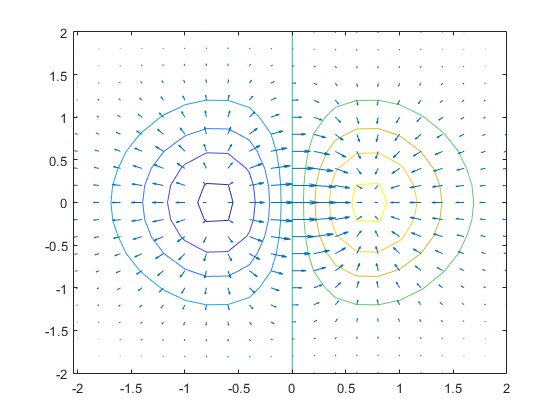如果把这个函数看做电势的函数，矢量图实际上就是电场线。
例子三：点电荷的电势：，简单化，假如，求电场线分布。
[X,Y] = meshgrid(-2:.2:2);
Z = 1./sqrt(X.^2 + Y.^2);
DX=-X./(X.^2+Y.^2).^(3/2);
DY=-Y./(X.^2+Y.^2).^(3/2);
figure;
quiver(X,Y,DX,DY);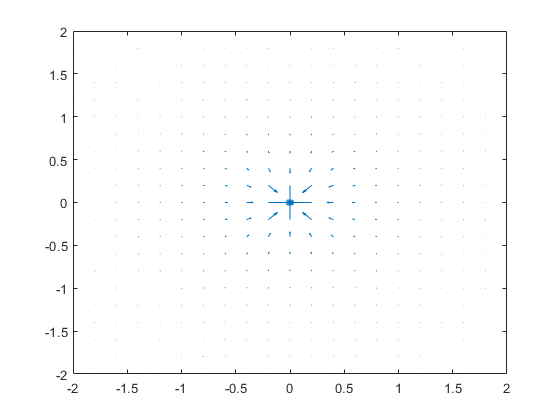Step 4:
LineStyle连接https://ww2.mathworks.cn/help/matlab/ref/linespec.html
展开全文• 1 2 3 4 5 6 7 8 9 10 11 12 13 14 15 16 17 18 19 20 21 22 23 24 25 26 27 28 29 30 31 32 33 34 35 36 37 38 39 40 41 42 43 44 45 46 %% =======绘制方向和速度矢量图======= %compass-绘制罗盘图 %feather-绘制...

1 2 3 4 5 6 7 8 9 10 11 12 13 14 15 16 17 18 19 20 21 22 23 24 25 26 27 28 29 30 31 32 33 34 35 36 37 38 39 40 41 42 43 44 45 46  %% =======绘制方向和速度矢量图======= %compass-绘制罗盘图 %feather-绘制羽毛图 %quiver-绘制二维箭头图 %quiver3-绘制三维箭头图  %绘制罗盘图 figure; wdir=[45 90 90 45 360 335 360 270 335 270 335 335]; knots=[6 6 8 6 3 9 6 8 9 10 14 12]; rdir=wdir*pi/180; [x,y]=pol2cart(rdir,knots);% 极坐标转化为直角坐标 compass(x,y); title('风向和风力') %绘制羽毛图 figure; alpha=90:-10:0; r=ones(size(alpha)); m=alpha*pi/180; n=r*10; [u,v]=pol2cart(m,n);% 极坐标转化为直角坐标 feather(u,v); title('羽毛图') %罗盘图和羽毛图的比较 figure; t=-pi/2:pi/12:pi/2; % 在 区间，每 取一点。 r=ones(size(t)); % 单位半径 [x,y]=pol2cart(t,r); % 极坐标转化为直角坐标 subplot(1,2,1),compass(x,y),title('Compass') subplot(1,2,2),feather(x,y),title('Feather') %绘制箭头图 figure; [x,y] = meshgrid(-2:.2:2,-1:.15:1); z = x .* exp(-x.^2 - y.^2);  [px,py] = gradient(z,.2,.15); subplot(1,2,1); contour(x,y,z), hold on quiver(x,y,px,py), hold off, axis image title('quiver示例'); [x,y,z]=peaks(15); [nx,ny,nz]=surfnorm(x,y,z);%surfnorm求平面的法向量 subplot(1,2,2) surf(x,y,z); hold on; quiver3(x,y,z,nx,ny,nz); title('quiver3示例');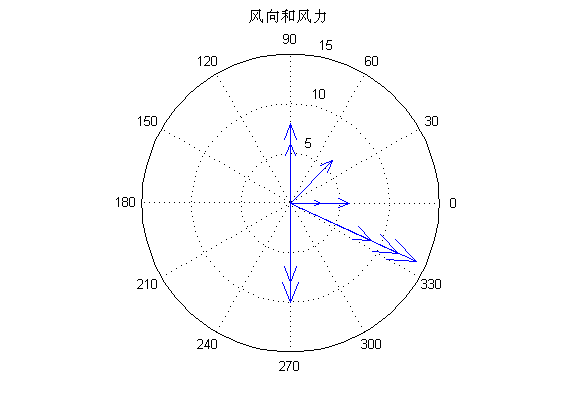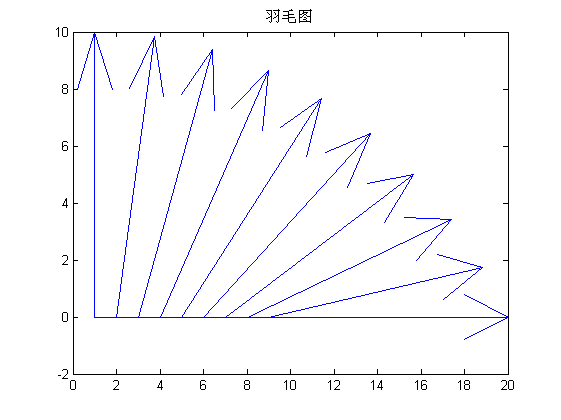展开全文• ## Matlab绘制矢量图

万次阅读 2009-07-28 11:53:00
经常会用到matlab计算矢量图，我们希望可以直观的... matlab提供了矢量绘制函数：quiver()matlab help给出了说明： QUIVER Quiver plot. QUIVER(X,Y,U,V) plots velocity vectors as arrows with components (u,v)
经常会用到matlab计算矢量图，我们希望可以直观的表示出来，通过观察来对比。
因此，希望可以绘制出矢量图。

matlab提供了矢量图绘制函数：quiver()
matlab help给出了说明：
QUIVER Quiver plot.    QUIVER(X,Y,U,V) plots velocity vectors as arrows with components (u,v)    at the points (x,y).  The matrices X,Y,U,V must all be the same size    and contain corresponding position and velocity components (X and Y    can also be vectors to specify a uniform grid).  QUIVER automatically    scales the arrows to fit within the grid.     QUIVER(U,V) plots velocity vectors at equally spaced points in    the x-y plane.     QUIVER(U,V,S) or QUIVER(X,Y,U,V,S) automatically scales the     arrows to fit within the grid and then stretches them by S.  Use    S=0 to plot the arrows without the automatic scaling.     QUIVER(...,LINESPEC) uses the plot linestyle specified for    the velocity vectors.  Any marker in LINESPEC is drawn at the base    instead of an arrow on the tip.  Use a marker of '.' to specify    no marker at all.  See PLOT for other possibilities.     QUIVER(...,'filled') fills any markers specified.     QUIVER(AX,...) plots into AX instead of GCA.     H = QUIVER(...) returns a quivergroup handle.     Backwards compatibility    QUIVER('v6',...) creates line objects instead of a quivergroup    object for compatibility with MATLAB 6.5 and earlier.       Example:       [x,y] = meshgrid(-2:.2:2,-1:.15:1);       z = x .* exp(-x.^2 - y.^2); [px,py] = gradient(z,.2,.15);       contour(x,y,z), hold on       quiver(x,y,px,py), hold off, axis image

例如要绘制[zx,zy]
zx 为x方向矢量
zy 为y方向矢量
(minx, maxx)为x方向取值区间
(miny, maxy)为y方向取值区间

[xx,yy]=meshgrid(minx:maxx, miny:maxy);quiver(xx,yy,zx,zy)

展开全文velocity components plot object
• 在搜索过程中，找到了MATLAB绘制海面风场图像，但实现过程中，显示出错 m_pcolor (line 43) data(i)=NaN。又是第一次接触matlab，有点儿懵。不过搞了一下午总算搞懂了，记录下菜鸡之路。 绘制的重点在于quiver函数...
在搜索过程中，找到了MATLAB绘制海面风场图像，但实现过程中，显示出错 m_pcolor (line 43) data(i)=NaN。又是第一次接触matlab，有点儿懵。不过搞了一下午总算搞懂了，记录下菜鸡之路。
绘制的重点在于quiver函数。

quiver(x,y,u,v)函数使用箭头来直观的显示矢量场。该调用格式表示通过在(x, y)指定的位置绘制小箭头来表示以该点为起点的向量(u,v)。 x、y、u、v的行数、列数必须对应相等。即x、y、u、v必须是同型矩阵。 需要注意的是，如果x、y不是矩阵，MATLAB会调用meshgrid函数将其扩展。然后再调用quiver函数。在这种情况下，x中元素的个数必须等于u、v的列数，y中元素个数必须等于u、v的行数（否则使用meshgrid扩展后无法满足x、y、u、v是同型矩阵，具体参见meshgrid函数）。

下载到的风场数据的（x,y,u,v）size如下
size(x)=73x144
size(y)=73x144
size(u)=144x73x31
size(v)=144x73x31 
对应的行列根本不相等！！！！
这是因为u和v是有31层（也就是z方向啦），所以要做的操作是将u和v的层级剥离出来，我们用在代码中的u和v应该只能是一层
剥离之后就简单了，代码如下
fname = '1.nc';
fnameu = 'C:\Users\2019HWL\PycharmProjects\data\u10\u-2.csv';
fnamev = 'C:\Users\2019HWL\PycharmProjects\data\v10\v-2.csv';
ncFilePath='1.nc';
[lo,la]=meshgrid(lon,lat);

figure
quiver(lo,la,uwind,vwind,3)
补充：
1、下载的是nc数据
2、剥离过程：

先用panoply（一个查看nc文件的软件）将u和v导出，导出的格式是csv的。
将上面得到的csv进行分层，也就是剥离过程。这是之前写的分层代码

来一张图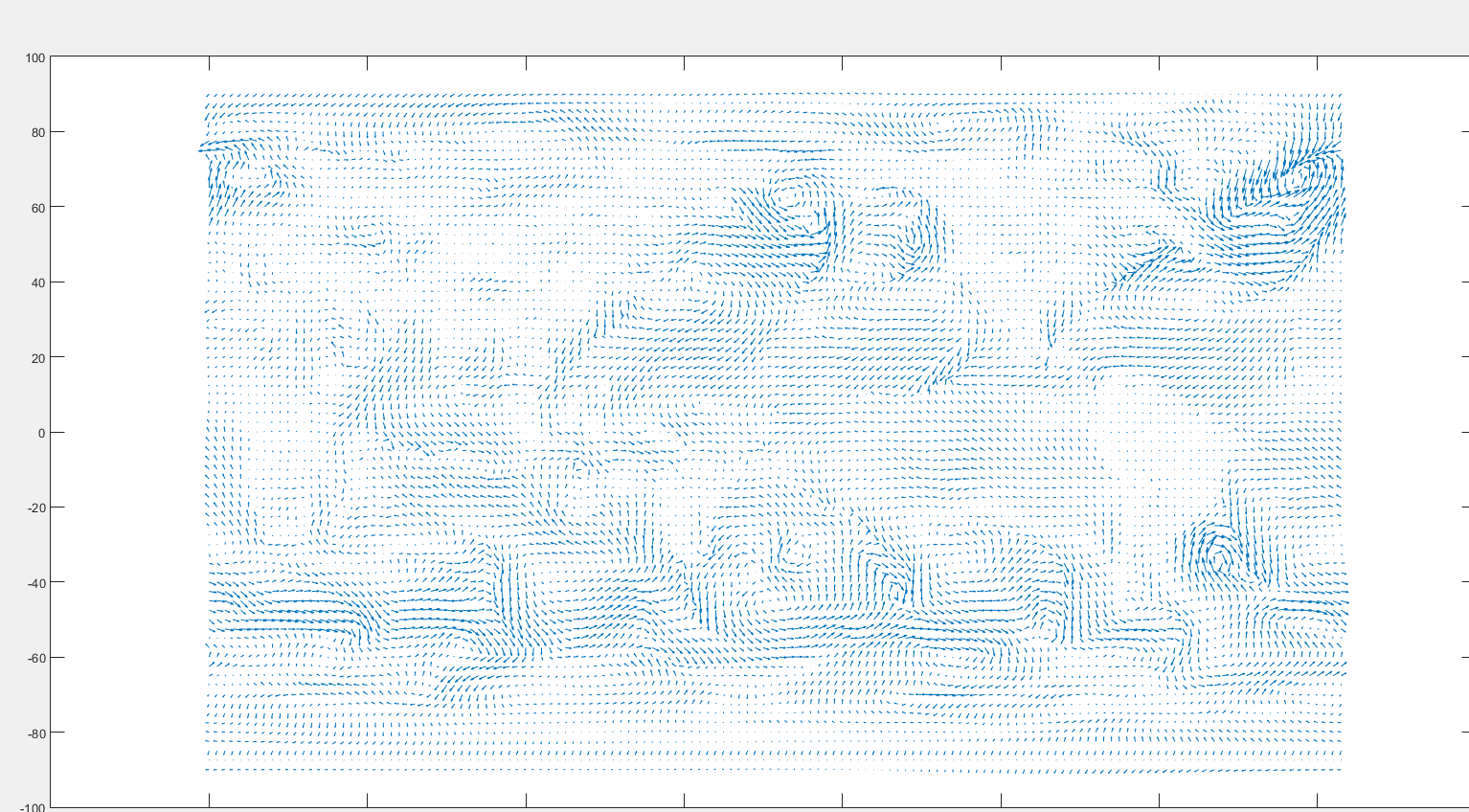展开全文• MATLAB矢量图，对整个矢量场进行插值，以箭头代表对应的方向，以颜色代表其大小。
• 1：文件-导出设置-渲染-向量格式 2：文件-导出设置-字体-time new（加粗，字体16磅）-应用于图窗 字体16磅-浏览，变成长方形，根据情况...九：MATLAB局部放大功能 方法一： Magnify： 1、需要在对应的文件夹中...
• 函数 VFIELD_COLOR 用颜色图定义的颜色绘制二维速度向量。 句法： VFIELD_COLOR(X,Y,U,V,SCALE,CMAP) 输入： X、Y 箭头原点、ND 阵列U、V 电流分量、ND 阵列SCALE 标量值设置矢量长度CMAP 颜色图，N x 3 RGB 值...
• MATLAB学习笔记（一）MATLAB矢量绘制quiver函数应用功能快捷键合理的创建标题，有助于目录的生成如何改变文本的样式插入链接与图片如何插入一段漂亮的代码片生成一个适合你的列表创建一个表格设定内容居中、居左、...
• 如果您正在绘制一些绝对场，如风矢量，则没有物理原因需要以这样的方式缩放矢量，即它们的区域分量在极点收缩为零。 除了解决上述问题外，quivermc还允许一些其他选项，包括颜色设置，箭头密度以及用于显示会聚或...
• VPLOT(X,Y,U,V) 将速度矢量绘制为彩色楔形（默认形状），方向由分量 (U,V) 和中心点 (X,Y) 定义。 数组 X、Y、U、V 的大小必须相同。 默认情况下，形状颜色由速度幅度决定。 VPLOT（X，Y，U，V，C）使用C确定形状...
• 用于分析标定误差区域提交算法精度，本资源上传原始图片和文档，供大家参考和学习标定中的问题定位方法。
• [X,Y] = meshgrid(1:256,1:256); [px,py] = gradient(rice_img_Gauss); quiver(X,Y,px,py) 放大后的效果
• PLOT_ALPHA(X,Y) 使用 Matlab FILL 绘制矢量 Y 与矢量 X。 包括三种方法： 1) 'fast' >> positive：一个宽度恒定的填充对象，问题：Kong2) 'fast2' >> 正：一个没有Kong的填充对象，问题：不同的宽度3) 'slow' >> ...
• MATLAB quiver函数使用：绘制矢量箭头介绍quiver()功能注意1：quiver()对(x,y,u,v)的要求注意2：quiver()绘制箭头时所在的坐标系举例总结代码及冰流速数据 介绍 在科研出图中，经常需要在底图上绘制流速图、受力图、...
• 1. 分别用mesh()和surf()绘制出函数xx.*exp(-xx.^2 - yy.^2)的例题曲面图。 例1（mesh）： x=linspace(-2, 2, 25); y=linspace(-2, 2, 25); [xx, yy]=meshgrid(x, y); zz=xx.*exp(-xx.^2 - yy.^2); mesh(xx, yy, zz)...梯度图
• ## MATLAB绘制向量图

千次阅读 2019-08-03 09:41:37
MATLAB绘制向量图 对于如何在二维平面中绘制带箭头的向量，先给出一个实例： clear; clc; A=[4,5]; B=[-10,0]; C=A+B; M=[A;B;C]; X=[M(:,1);A(1)]; Y=[M(:,2);A(2)]; plot(X,Y,'r'); axis ...
• CURVVEC 为二维矢量分量 U、V 创建曲线矢量。 数组 X、Y 定义了 U 和 V 的坐标。向量的曲率基于 ML 内置函数 STREAM2。 矢量的长度被缩放到矢量幅度。 根据可选的输入参数，矢量的颜色可能会或可能不会根据矢量幅度...
• 这时候可以使用matlab绘制显示 命令 这里x,y,z是点的位置 这里u,v,w是法线的位置 quiver3(x, y, z, u, v, w); demo： clc close all clear all [V,F,UV,TF,N,NF] = readOBJ('000001.obj'); x = V(:,1); y = V(:,2);...法线
• ## MatLab绘制图像

万次阅读 多人点赞 2018-08-26 23:43:06
title('梯度场矢量'); xlabel('x轴'); ylabel('y轴'); 图1.9 梯度场矢量图 2 三维图像 2.1 三维线型图 plot3函数 figure(12) n=20; [x12,y12,z12]=sphere(n); plot3(x12,y12,z12); grid on title('...二维
• % quiver(x,y,u,v) ...% 例如绘制f(x,y)=y-3x-2x^2-3xy-3y^2的方法： % 先用gradient函数计算偏导数，然后再用quiver绘制向量场 f=@(x,y) -y-3*x-2*x.^2-3*x.*y-y.^2; [x,y]=meshgrid(-20:0.5:20,-1...
• 用法plot_vectors(向量) plot_vectors(vectors,normalize) 输入- VECTORS：在单元 i 中具有 Vix2 或 Vix3 矩阵 (i=1,2,...) 的单元阵列，以及 Vi 向量的 x、y 和 z 坐标- NORMALIZE: 1 (归一化向量) 或 0 (不归一化...
• 这个 MATLAB 工具制作具有纸张质量的平行坐标图。 平行坐标图允许高维数据的可视化。 实现了以下功能： * 过滤和排序变量* 缩放变量范围* 找出构成情节的线条的颜色* 提取并突出显示特殊行* 绘制不同变量的范围 该...
• 翻译自 MATLAB 官方文档 feather : 羽毛图，绘制速度向量 quiver : 箭头图 compass : 罗盘图 quiver3 : 3D 箭头图 streamslice : 在切片平面中绘制流线图 streamline : 根据二维或三维向量数据绘制流线图 ...
• 1 MATLAB 大作业 用MATLAB 对椭圆偏振光矢量末端的三维轨迹进行仿真 要求: Ex=cos(wt+a1 Ey=cos(wt+a2 t 取一个半周期左右 m=a1-a1 做出m=0, pi/2, -pi/2, pi/4, -pi/4 时的轨迹图;区别分左/右旋;并与理论作对比,对...
• DARROW 在指定方向的指定点绘制箭头 DARROW(X,Y,U,V,S) 对于标量参数，在方向 (U,V) 上的点 (X,Y) 处绘制长度为 S 的箭头。 当一个或多个输入参数为列向量时，在指定方向的每一点绘制指定长度的箭头 H = DARROW(......
• 今天博主跟大家讲一下如何用MATLAB制作一幅中国地图，那废话不多说，我们先看一下最终效果吧。 mercator墨卡托圆柱投影地图    lambert兰伯特圆锥投影地图  一张中国地图大概包括以下要素： 中国...
• matlab 绘制三维空间的立体箭头 function arrow3(X1,X2,r1,h,r2,color) %一个简单的实例：arrow3([1 2 3],[7 8 9],0.25,1,0.6,'b'); %箭头由圆柱体和圆锥体组成:包括圆柱体的起点和终点位置，以及半径，圆锥的半径...
• MATLAB绘制系统根轨迹和性能分析一、练习目的熟悉MATLAB用于控制系统中的一些基本编程语句和格式。利用MATLAB语句绘制系统的根轨迹。掌握用根轨迹分析系统性能的图解方法。掌握系统参数变化对特征根位置的影响。二...
• 1、海面风场数据下载 从ECNWF... 以下为可以下载的参数数据，选择10 metre U wind Component和10 metre V wind Component（这里我们下载的是.nc格式的） 2、MATLAB安装m_map工具箱 https://www.......

# matlab绘制矢量matlab 订阅